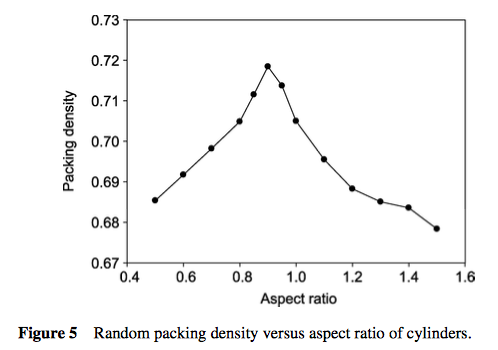# Random packing density of cylinders in a volume

I am trying to calculate the packing density of cylindrical bottles in a box, assuming that the bottles are randomly dumped in the box.

I have read on the packing density of spheres here https://en.wikipedia.org/wiki/Random_close_pack but have found nothing on cylinders.

For example, if I randomly dump bottles with a diameter of 75 mm and a height of 145 mm in a volume of 0.6 cubic meters, what would be the packing density (the end goal is to calculate how many bottles could approximately fit in such a space).

• You might like to use the discrete geometry tag since a significant part of discrete geometry deals with packing problems. – user62562 Nov 7 '16 at 20:18
• Right. This is definitely not algebraic geometry. Possible relevant tags are probability, geometric measure theory. – Anthony Quas Nov 7 '16 at 20:46

## 2 Answers

An overview of results for the random packing densities of basic 3D objects, including cylinders, is given in this article (2010). Here is the plot for cylinders as a function of aspect ratio (height over diameter):The peak value of 0.72 is reached for an aspect ratio of 0.9. These are numerical results. The maximum (rather than random) packing density of cylinders is the densest ordered packing of circles in 2D, which is $$(\pi/6)\sqrt 3=0.9069$$.

• Is the graph plotting the highest packing density achieved over an ensemble of random packings? (Unfortunately the reference is a bit short on methodology.) – Nawaf Bou-Rabee Nov 8 '16 at 13:03
• @NawafBou-Rabee --- that is how understand the statement that $0.72$ is the "upper bound of the packing density for a random packing of cylinders" – Carlo Beenakker Nov 8 '16 at 13:33

Your problem seems extremely difficult to approach rigorously. There is always the experimental approach, most efficiently by computer simulation. Even the problem of the average density of random packing of congruent circles in the plane is, to my knowledge, still unsolved, though computer simulation is not so hard (see http://www.sciencedirect.com/science/article/pii/0021979771903389 ).